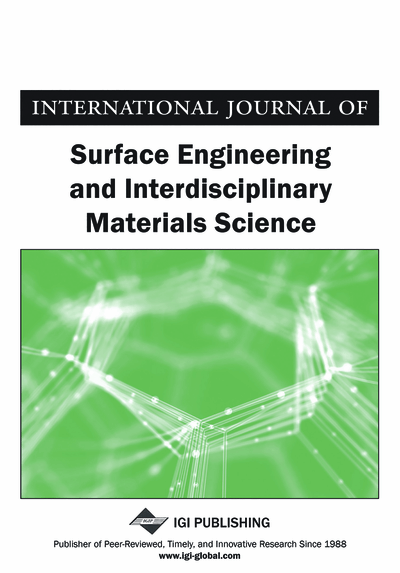# Hydrodynamic Behavior of the Sliding Surface with Semicircular Pores: Theoretical and MATLAB Considerations

Leonid Burstein (Kinneret Academic College, Tzamah, Israel)
DOI: 10.4018/IJSEIMS.2016010103
Available
\$37.50
No Current Special Offers

## Abstract

A mathematical model, theoretical and numerical solutions are represented for non-contacting sliding surfaces with pores having a half-round form. The theoretically based expressions for the hydrodynamic pressure, the maximum positive pressure and the load capacity were obtained for a cell which includes the inside and outside of the pore areas. The cell and pore dimensions were studied in the range 7.5…100 and 5... 20 micron respectively. The obtained expressions were validated with the MATLAB ODE-solver. It is shown that behavior of the sliding surfaces with semicircular pores can be achieved for the pores with pore radii-gap ratio about 0.5 … 2 and the control cell dimensions to pore radii ratios about 3 … 5. The rate of performance improvement becomes small above these values. In general, the optimum pore size decreases at higher pore cell-pore ratios and lower pore-gap ratio values.
Article Preview
Top

## 2. Model And Basic Equations

Cross-section of the two opposite surfaces with hemispherical pores on the lower surface are shown in Fig.1; each pore has radius r0 and the gap between surfaces equals h0.

Assuming that the pores are arranged regularly and the distance 2r1 between pores (see Figure 1) is sufficiently large so that the interaction between the pores can be neglected, only one pore with the adjoining part, called the control cell, can be studied. The hydrodynamic lubrication is described by the Reynolds equation, which in its dimensionless form is

(1) where

• X=x/r0,

• H=h/h0,

• P=p/Λ,

• Λ=6μur0/h02.

Here and hereinafter:

• P = p/Λ - dimensionless hydrodynamic pressure;

• p - dimensional hydrodynamic pressure;

• u- dimensional velocity of sliding surface, along coordinate x;

• r0 - dimensional pore radii;

• r1 – control cell dimension;

• h0 - dimensional minimal clearance (gap) between surfaces;

• X=x/r0 - dimensionless coordinate;

• x - dimensional coordinate;

• H – dimensionless local film thickness;

• h – dimensional local film thickness;

• W=w/(Λr0) - dimensionless load support;

• Λ=6μur0/h02;

• μ - dimensional dynamic viscosity of lubricating film;

• w- dimensional load support along x-direction;

• Ψ= r0/ h0 - pore radii to gap value ratio;

• ξ = r1/r0 – control cell dimension to pore radii ratio;

• max – maximal value;

• cav – cavitation threshold.

## Complete Article List

Search this Journal:
Reset
Open Access Articles: Forthcoming
Volume 10: 2 Issues (2022): 1 Released, 1 Forthcoming
Volume 9: 2 Issues (2021)
Volume 8: 2 Issues (2020)
Volume 7: 2 Issues (2019)
Volume 6: 2 Issues (2018)
Volume 5: 2 Issues (2017)
Volume 4: 2 Issues (2016)
Volume 3: 2 Issues (2015)
Volume 2: 2 Issues (2014)
Volume 1: 2 Issues (2013)
View Complete Journal Contents Listing